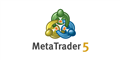# mql5 standard deviation channel formula code -good coder wanted

Hi all,

does any one know what formula is used in creating the mql5 standard deviation channels object OBJ_STDDEVCHANNEL and how this formula can be coded from first principles in mql5 to derive the channel values.

I think this could be a freelance opportunity for some one with this knowledgeStandard Deviation - Trend Indicators - MetaTrader 5 Help
Standard Deviation — value of the market volatility measurement. This indicator describes the range of price fluctuations relative to Moving...

```      // Standard deviation - //en.wikipedia.org/wiki/Standard_deviation
// SMA = 1/n sum(X)
// Std = Sqrt[1/n sum{(x-sma)^2}] = Sqrt[1/n Sum(x^2)-(1/n Sum(x))^2]
double   sumX  = 0.0, sumX2   = 0.0;
for(INDEX i=iBar+agkLength; i>iBar;){  --i;
CHANGE   tr = TRD(i);   sumX += tr; sumX2 += tr * tr;
}
double      sma   = sumX / agkLength,
std   = MathSqrt(sumX2/agkLength - atr*atr);
```

William Roeder #:

Thanks William,

I will work on this.

Its a pity that the standard deviation channel values are not directly calculable in mql5 as they are for so many other indicators such as the MA functions.